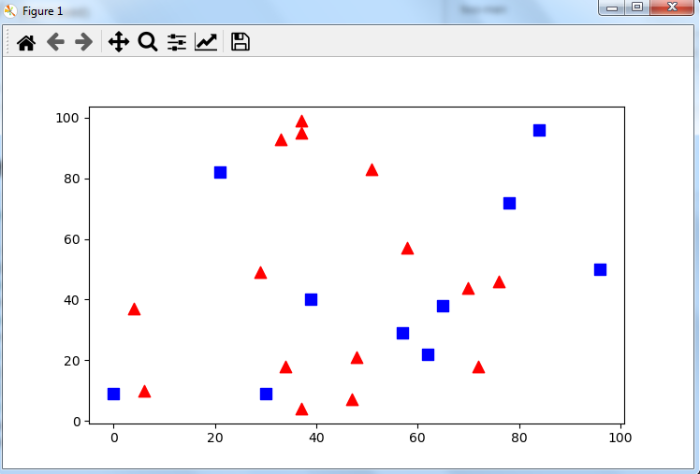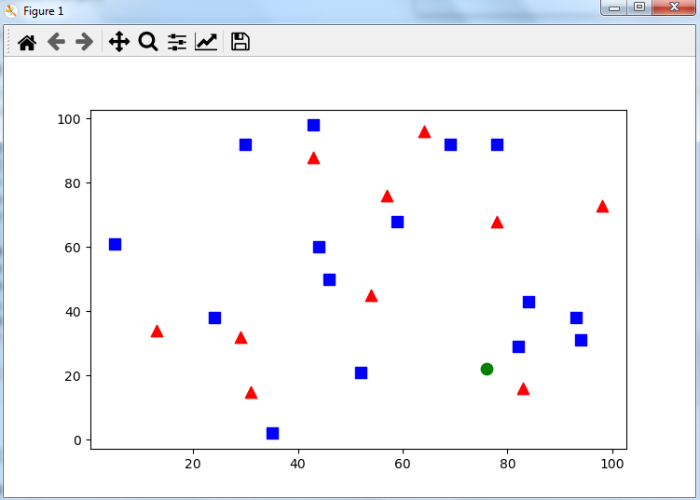# Implementing k-Nearest Neighbor in OpenCV Python?

k-Nearest Neighbor (kNN) is a simple classification algorithm for supervised learning. To implement kNN in OpenCV, you can follow the steps given below −

• Import the required libraries OpenCV, NumPy and Matplotlib.

• We define two classes Red and Blue each having 25 numbers. Then generate training data for these two classes using a random generator.

• Next, we generate the Labels for each training data. The label for Red family numbers is 0 and for Blue family members is 1.

• Now plot the Red and Blue family members.

• Generate a new number using the random generator and plot it.

• Initiate a KNearest object knn and train it with training data.

• Finally, compute the knn.findNearest() for the new number to find the class label of the new member, class labels and distances of nearest neighbors.

Let's have a look at the examples below to implement the k-Nearest Neighbors.

## Example

In this example, we generate and plot 25 training data for both red and blue families.

# import required libraries
import cv2
import numpy as np
import matplotlib.pyplot as plt

# Feature set containing (x,y) values of 25 known/training data
trainData = np.random.randint(0,100,(25,2)).astype(np.float32)

# Labels each one either Red or Blue with numbers 0 and 1
responses = np.random.randint(0,2,(25,1)).astype(np.float32)

# Take Red families and plot them
red = trainData[responses.ravel()==0]
plt.scatter(red[:,0],red[:,1],80,'r','^')

# Take Blue families and plot them
blue = trainData[responses.ravel()==1]
plt.scatter(blue[:,0],blue[:,1],80,'b','s')
plt.show()


## Output

When you run the above Python program, it will produce the following output window −## Example

In this example, we generate and plot 25 training data for both red and blue families. Now we generate a new number and apply the K-nearest neighbor algorithm to classify the new number to the red or blue family.

# import required libraries
import cv2
import s numpy as np
import matplotlib.pyplot aplt

# Feature set containing (x,y) values of 25 known/training data
trainData = np.random.randint(0,100,(25,2)).astype(np.float32)

# Labels each one either Red or Blue with numbers 0 and 1
responses = np.random.randint(0,2,(25,1)).astype(np.float32)

# Take Red families and plot them
red = trainData[responses.ravel()==0]
plt.scatter(red[:,0],red[:,1],80,'r','^')

# Take Blue families and plot them
blue = trainData[responses.ravel()==1]
plt.scatter(blue[:,0],blue[:,1],80,'b','s')

# take new point
newcomer = np.random.randint(0,100,(1,2)).astype(np.float32)
plt.scatter(newcomer[:,0],newcomer[:,1],80,'g','o')
knn = cv2.ml.KNearest_create()
knn.train(trainData, cv2.ml.ROW_SAMPLE, responses)
ret, results, neighbors ,dist = knn.findNearest(newcomer, 3)
print("Label of New Member: {}\n".format(results) )
print("Nearest Neighbors: {}\n".format(neighbors) )
print("Distance of Each Neighbor: {}\n".format(dist) )
plt.show()


## Output

When you run the above Python program, it will produce the following output

Label of New Member: [[1.]]
Nearest Neighbors: [[0. 1. 1.]]
Distance of Each Neighbor: [[ 85. 85. 405.]]


The result shows that the new number belongs to the Blue family as two of the three nearest neighbor belong to the blue family. It will also display the following output window −